• 1.向量加法 LearnOpenGL - Transformations
1.向量加法
LearnOpenGL - Transformations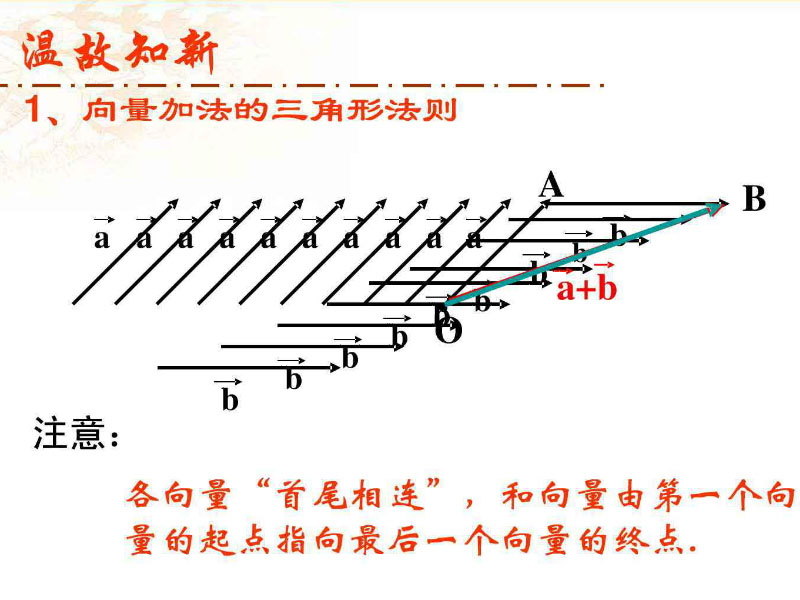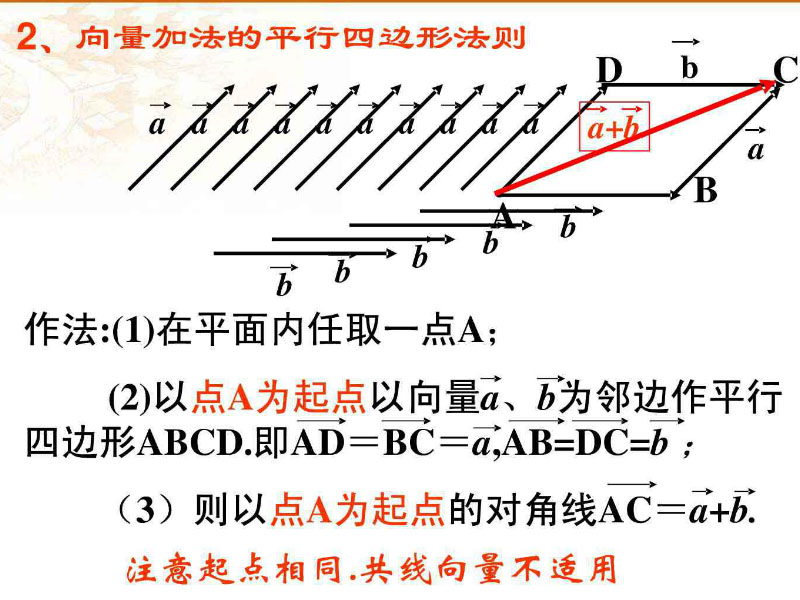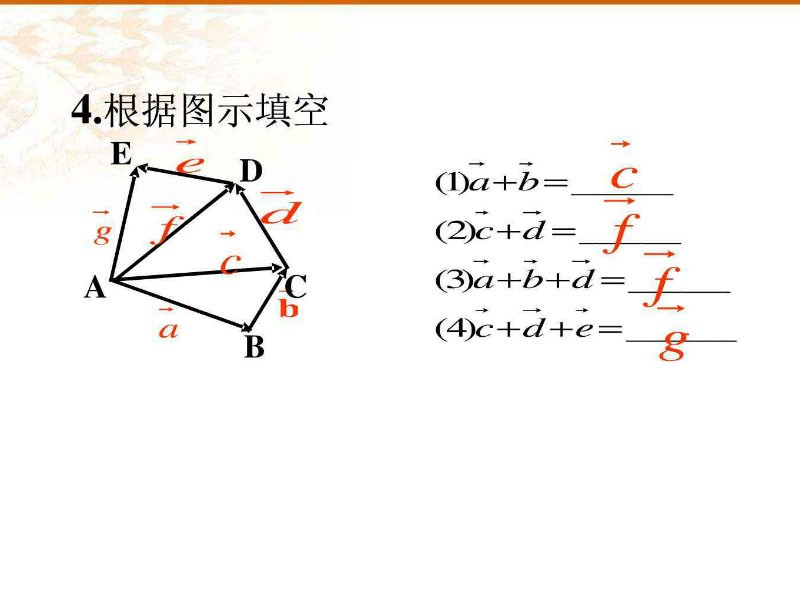向量加法举栗子，使用LearnOpenGL书山的例子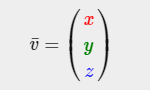向量的加法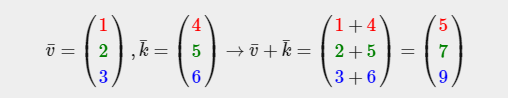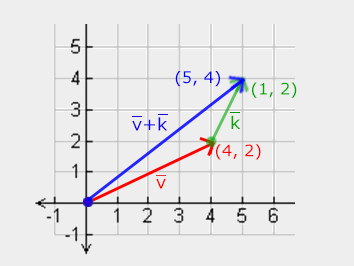2.向量减法
减法应该就是把方向反过来，做加法而已，
注意是b的终点到a的终点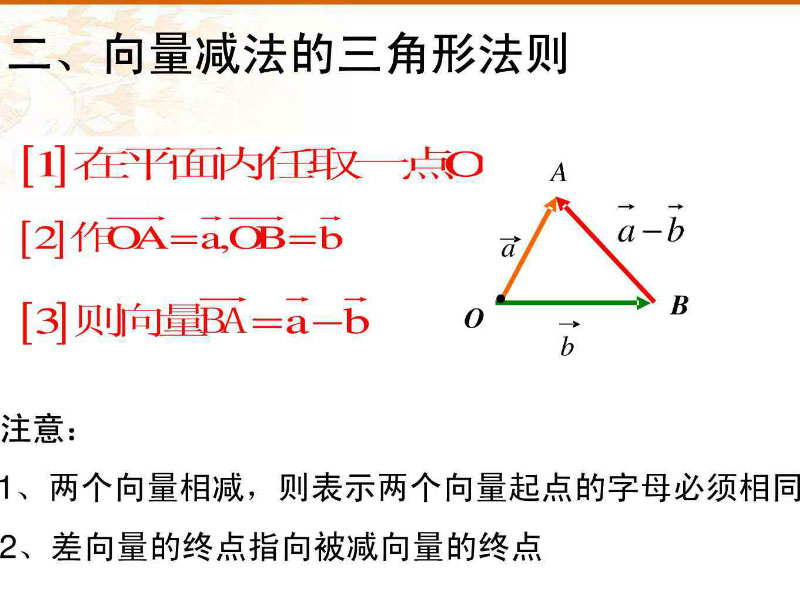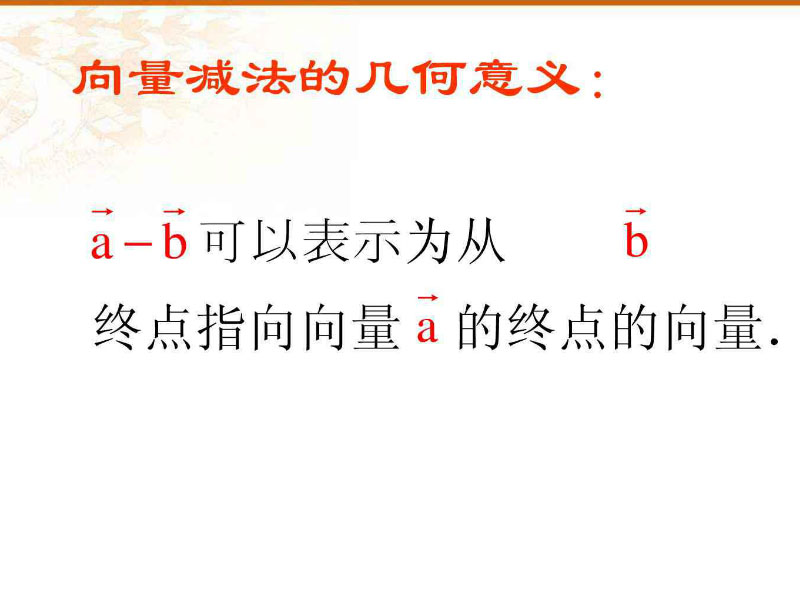向量减法举栗子，还使用LearnOpenGL书山的例子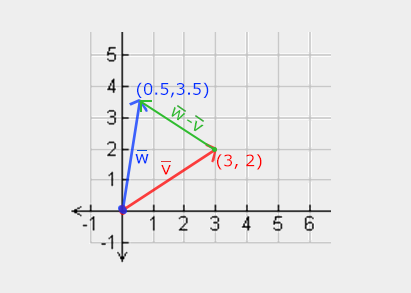向量的长度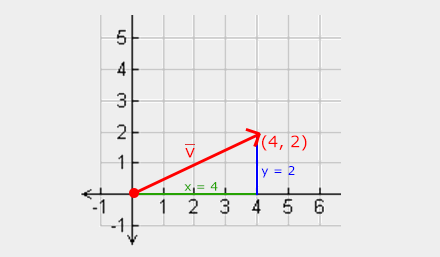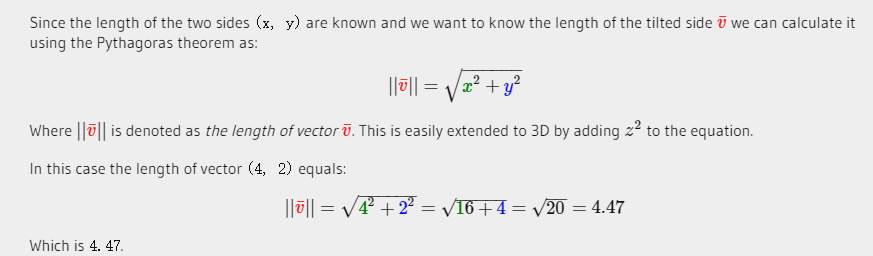向量归一化(变成单位向量)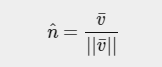3.向量的点乘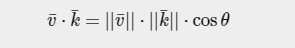如果2个都是单位向量时：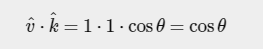当角度为90度时，余弦或cos函数变为0，而当角度为0时，余弦或cos函数变为1。这使我们可以使用点积轻松地测试两个向量是正交还是平行（正交表示 向量彼此成直角）。
如下是2个单位向量的点积计算： cosθ=-0.8,可计算出角度为θ=143.1°    请看这：反正弦、反余弦和反正切 (shuxuele.com)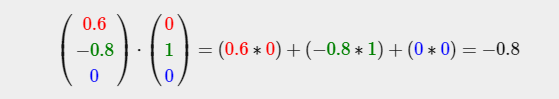点乘的几何意义是可以用来表征或计算两个向量之间的夹角，以及在b向量在a向量方向上的投影，
4.向量的叉乘
叉积仅在3D空间中定义，并采用两个非平行向量作为输入，并产生与两个输入向量正交的第三向量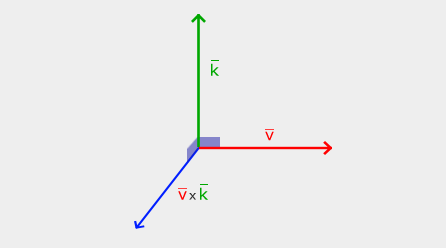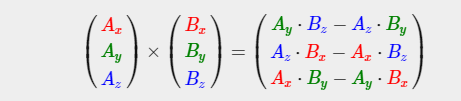叉乘的几何意义：在三维几何中，向量a和向量b的叉乘结果是一个向量，更为熟知的叫法是法向量，该向量垂直于a和b向量构成的平面。

5.向量除法
我们先来看看什么是除法——已知两个因数的积与其中一个因数，求另一个因数的运算，叫做除法。除法可以看成是“乘法的逆运算”．
所以，简单的说，逆运算就是在求逆元。
而我们知道的向量的乘法有两种，一个是数量积数量积(点乘)，一个是向量积向量积（叉乘）。
·先来看看数量积有没有逆运算呢？ 如果数量积有除法的话，设向量和的乘积为(数)，即那么
如果数量积有除法的话，设向量a和b的乘积为x(数)，即a.b=x 那么x/a=b由数量积的定义， 两个向量的数量积等于一个向量的模乘以另一个向量在此向量上的投影，那么如果a确定的话， 改变b的方向和大小，发现有无数个向量的投影等用于b在a方向上的投影，即如果乘积不变， 则向量b的解是无穷多的，所以向量的商不是唯一确定的。所以数量积的逆运算是没有的╮(╯_╰)╭大概看图就这样理解吧……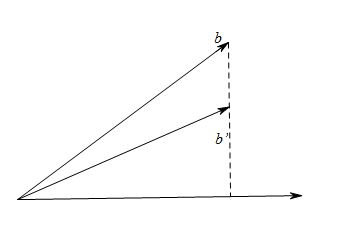对于高中的小朋友讲完数量积其实就可以差不多告一段落了~
但是还有向量积呢……
那再来看看向量积有没有逆运算呢？我也继续假设向量积存在除法，因为向量积的结果仍然是一个向量， 设向量a和b的乘积为x(向量)，即a*b=x那么x/a=b我们知道，向量积的模可以看作平行四边形的面积，那么如果a确定的话， 那么变化x的长度和方向，也可以得到相同面积的平行四边形，显然向量b的解是无穷多的， 所以向量的商不是唯一确定的。所以数量积的逆运算还是没有的╮(╯_╰)╭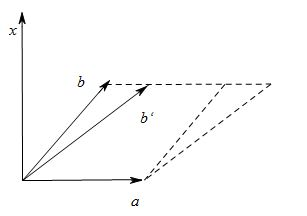我们发现对于数量积和向量积，他们的逆运算都是不确定的，所以，向量的除法是不存在的.

其实学了群以后，就不用这么繁琐的讲这么多了。


展开全文opengl
• Vector arithmetic is fundamental to 3D graphics, physics and animation and it is useful to understand it in depth to get the most out of Unity. Below are descriptions of the main operations and some ...
Vector arithmetic is fundamental to 3D graphics, physics and animation and it is useful to understand it in depth to get the most out of Unity. Below are descriptions of the main operations and some suggestions about the many things they can be used for. \
向量运算是三维图形，物理和动画的基础，对它深入了解，对Unity非常有帮助。下面是一些主要运算的描述,可用于对很多事情的描述和建议。
When two vectors are added together, the result is equivalent to taking the original vectors as "steps", one after the other. Note that the order of the two parameters doesn't matter, since the result is the same either way.
当两个向量相加，是体格向量的头到另一个向量的尾，一前一后。请注意，这两个参数的顺序并不重要，因为无论哪种方式结果是相同的。

If the first vector is taken as a point in space then the second can be interpreted as an offset or "jump" from that position. For example, to find a point 5 units above a location on the ground, you could use the following calculation:-
如果第一个向量空间中的点，那么第二个可以解释为从这个点位移或"跳跃" 。例如，要找到地面上的某个点5个以上的单位的位置，你可以使用以下的计算
var pointInAir = pointOnGround + new Vector3(0, 5, 0);
If the vectors represent forces then it is more intuitive to think of them in terms of their direction and magnitude (the magnitude indicates the size of the force). Adding two force vectors results in a new vector equivalent to the combination of the forces. This concept is often useful when applying forces with several separate components acting at once (eg, a rocket being propelled forward may also be affected by a crosswind).
如果向量代表的力，那么可以更直观的表示为在他们自己的方向上的力（长度表示力的大小）。两个力相加的结果是这两个力的合力。这个概念经常用到，当几个分利同时执行的时候（例如，推动火箭也可能受侧风的影响）。
Subtraction 减法
Vector subtraction is most often used to get the direction and distance from one object to another. Note that the order of the two parameters does matter with subtraction:-
向量减法经常用来计算一个对象从一个点到另一个的方向和距离。请注意，这两个参数的顺序与减法的问题：

// The vector d has the same magnitude as c but points in the opposite direction. var c = b - a; var d = a - b;
As with numbers, adding the negative of a vector is the same as subtracting the positive.
类似于数字计算，加上一个负的等于减去一个正的。
// These both give the same result. var c = a - b; var c = a + -b;
The negative of a vector has the same magnitude as the original and points along the same line but in the exact opposite direction.
负的向量跟正的向量在同一条直线上 并且方向相反。
Scalar Multiplication and Division 标量乘法和除法
When discussing vectors, it is common to refer to an ordinary number (eg, a float value) as a scalar. The meaning of this is that a scalar only has "scale" or magnitude whereas a vector has both magnitude and direction.
当我们讨论的向量，它常用他的标量作为一个普通的数字（例如，一个float值） 。这表示标量只有大小，而向量的大小和方向。
Multiplying a vector by a scalar results in a vector that points in the same direction as the original. However, the new vector's magnitude is equal to the original magnitude multiplied by the scalar value.
向量乘以一个标量方向和位置仍为原来的方向和位置。然而，新的向量的大小等于原来的大小乘以标量。
Likewise, scalar division divides the original vector's magnitude by the scalar.
同样，标量的除法结果就是标量的几分之一。
These operations are useful when the vector represents a movement offset or a force. They allow you to change the magnitude of the vector without affecting its direction.
向量代表一个移动或力时，这些运算是非常有用的。他们允许你改变向量的大小而不影响其方向。
When any vector is divided by its own magnitude, the result is a vector with a magnitude of 1, which is known as a normalized vector. If a normalized vector is multiplied by a scalar then the magnitude of the result will be equal to that scalar value. This is useful when the direction of a force is constant but the strength is controllable (eg, the force from a car's wheel always pushes forwards but the power is controlled by the driver).
任何向量除以他自己的大小，其结果是一个长度为1的向量，这被称为单位向量。如果一个单位向量乘以一个标量，那么结果的长度将标量的大小。当力的方向是不变的，但力是可控的时.这是非常有用的.（例如，一辆车的车轮的力总是向前的，但力的大小是由司机控制） 。
Dot Product 点乘
The dot product takes two vectors and returns a scalar. This scalar is equal to the magnitudes of the two vectors multiplied together and the result multiplied by the cosine of the angle between the vectors. When both vectors are normalized, the cosine essentially states how far the first vector extends in the second's direction (or vice-versa - the order of the parameters doesn't matter).
两个向量想成的结果是一个标量。此标是等于两个向量长度相乘结果乘以向量之间的夹角的余弦。当两个向量都为单位向量时，余弦的定义就表示为第一个向量在第二个向量上面的射影长度（或反之亦然 - 参数的顺序并不重要） 。

It is easy enough to think in terms of angles and then find the corresponding cosines using a calculator. However, it is useful to get an intuitive understanding of some of the main cosine values as shown in the diagram below:-
容易想像，在角度方面可以通过计算器计算余弦值。然而，下面图标中的一些主要的余弦值是会经常用到的。

The dot product is a very simple operation that can be used in place of the Mathf.Cos function or the vector magnitude operation in some circumstances (it doesn't do exactly the same thing but sometimes the effect is equivalent). However, calculating the dot product function takes much less CPU time and so it can be a valuable optimization.
点程是一个非常简单的操作，可以在需要矢量长度操作的地方用Mathf.Cos功能（它不会做同样的事情，但有时效果是等价的）。尽管如此,计算点乘功能需要CPU时间要少得多，因此它可以是一个有价值的优化。
Cross Product 叉乘
The other operations are defined for 2D and 3D vectors and indeed vectors with any number of dimensions. The cross product, by contrast, is only meaningful for 3D vectors. It takes two vectors as input and returns another vector as its result.
其他操作被定义为二维和三维向量和任意维数向量。叉乘只能用来计算3D向量，它需要输入两个向量返回结果是另一个向量。
The result vector is perpendicular to the two input vectors. The "left hand rule" can be used to remember the direction of the output vector from the ordering of the input vectors. If the first parameter is matched up to the thumb of the hand and the second parameter to the forefinger, then the result will point in the direction of the middle finger. If the order of the parameters is reversed then the resulting vector will point in the exact opposite direction but will have the same magnitude.
得到的结果垂直于输入的两个向量。"左手坐标系"可以用来表示输入和输出的向量的方向。如果第一个参数匹配手的拇指和食指匹配第二个参数，结果将是中指的方向。如果参数的顺序是相反的结果向量将指向正好相反的方向，但将有相同长度。

The magnitude of the result is equal to the magnitudes of the input vectors multiplied together and then that value multiplied by the sine of the angle between them. Some useful values of the sine function are shown below:-
结果的大小等于输入向量的乘积，然后通过它们之间的角度的正弦值乘以该值的大小。

The cross product can seem complicated since it combines several useful pieces of information in its return value. However, like the dot product, it is very efficient mathematically and can be used to optimize code that would otherwise depend on slow transcendental functions.
叉乘比较复杂，因为在其返回值结合了一些有用的信息。但是像点乘那样,这些非常有效的数学可以用来优化代码，否则将依赖于缓慢的超越函数。

举个栗子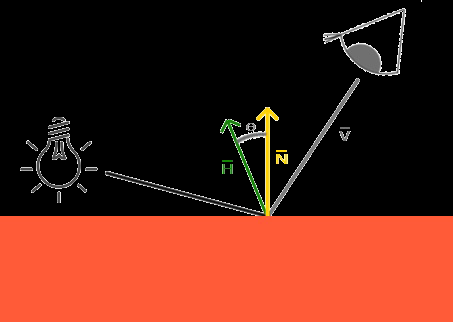#version 450 core

out vec4 FragColor;

in VS_OUT
{
vec3 FragPos;     //传入地面在世界空间中的坐标
vec3 Normal;
vec2 TexCoords;
} fs_in;

uniform sampler2D floorTexture;
uniform vec3 lightPos;                //光源位置
uniform vec3 viewPos;                 //观察者位置
uniform bool blinn;                   //是否是blinn-phong光照模型

void main()
{
vec3 color = texture(floorTexture, fs_in.TexCoords).rgb;
//ambient
vec3 ambient = 0.05 * color;     //环境光照分量
//diffuse
//向量减法经常用来计算一个对象从一个点到另一个的方向和距离。请注意，这两个参数的顺序与减法的问题
vec3 lightDir = normalize(lightPos - fs_in.FragPos);   //光源位置到地面位置的距离
vec3 normal = normalize(fs_in.Normal);
float diff = max(dot(lightDir, normal), 0.0f);
vec3 diffuse = diff * color;     散射光照分量
//specular
//观察者位置到地面位置的距离
vec3 viewDir = normalize(viewPos - fs_in.FragPos);
vec3 reflectDir = reflect(-lightDir, normal);
float spec = 0.0f;
if(blinn)   //blinn-phong光照模型
{
vec3 halfwayDir = normalize(lightDir + viewDir);
spec = pow(max(dot(normal, halfwayDir), 0.0f), 32.0f);  //镜面光照分量
}
else
{
vec3 reflectDir = reflect(-lightDir, normal);
spec = pow(max(dot(viewDir, reflectDir), 0.0f), 8.0f);  //phong光照模型
}

vec3 specular = vec3(0.3) * spec; // assuming bright white light color
FragColor = vec4(ambient + diffuse + specular, 1.0f);

}
运行效果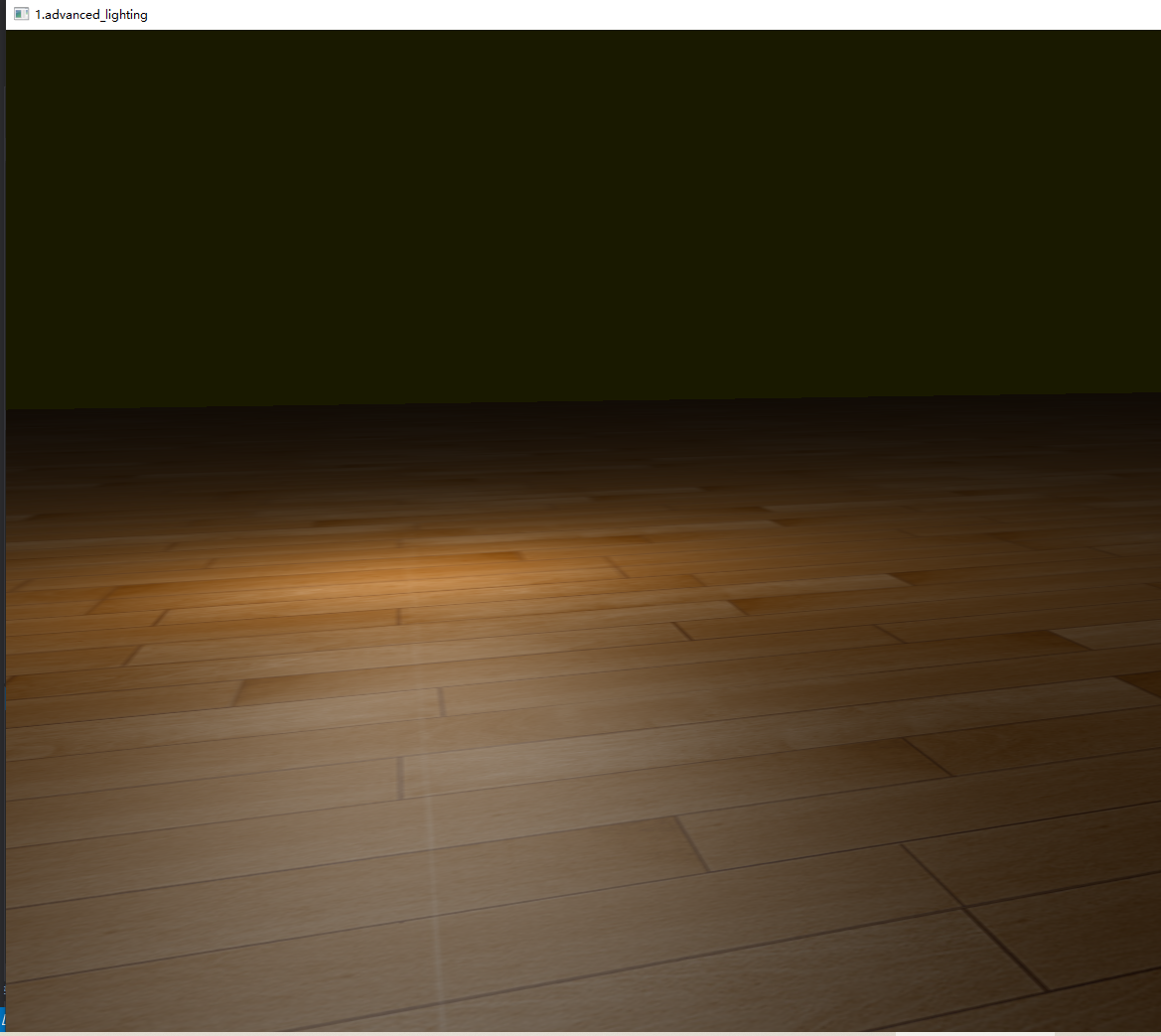源码下载
展开全文• 文章目录matlab 矩阵加减乘除运算1 加、减运算2 乘法**3．向量点积****4．向量叉乘****5．混合积****6．矩阵的卷积和多项式乘法**7．反褶积（解卷）和多项式除法运算8．张量积**9 除法运算** matlab 矩阵加减乘除...


文章目录
matlab 矩阵加减乘除运算1 .加、减运算2. 乘法3．向量点积4．向量叉乘5．混合积6．矩阵的卷积和多项式乘法7．反褶积（解卷）和多项式除法运算8．张量积9. 除法运算

matlab 矩阵加减乘除运算
1 .加、减运算
运算符：“＋”和“－”分别为加、减运算符。 运算规则：对应元素相加、减，即按线性代数中矩阵的“十”，“一”运算进行。
2. 乘法
运算符：* 运算规则：按线性代数中矩阵乘法运算进行，即放在前面的矩阵的各行元素，分别与放 在后面的矩阵的各列元素对应相乘并相加。
3．向量点积
函数 dot 格式 C = dot(A,B) %若 A、B 为向量，则返回向量 A 与 B 的点积，A 与 B 长度 相同；若为矩阵，则 A 与 B 有相同的维数。 C = dot(A,B,dim) %在 dim 维数中给出 A 与 B 的点积
例 >>X=[-1 0 2]；
>>Y=[-2 -1 1];
>>Z=dot(X, Y)
则显示：Z =
4
还可用另一种算法：
sum(X.*Y)
ans=
4

4．向量叉乘
在数学上，两向量的叉乘是一个过两相交向量的交点且垂直于两向量所在平面的向量。 在 Matlab 中，用函数 cross 实现。 函数 cross 格式 C = cross(A,B) %若 A、B 为向量，则返回 A 与 B 的叉乘，即 C=A×B，A、B 必须是 3 个元素的向量；若 A、B 为矩阵，则返回一个 3×n 矩阵，其中的列是 A 与 B 对应列的叉积，A、B 都是 3×n 矩 阵。 C = cross(A,B,dim) %在 dim 维数中给出向量 A 与 B 的叉积。A 和 B 必须具有 相同的维数，size(A,dim)和 size(B,dim)必须是 3。 例 1-24 计算垂直于向量(1, 2, 3)和(4, 5, 6)的向量。
 >>a=[1 2 3];
>>b=[4 5 6];
>>c=cross(a,b)
结果显示：
c=
-3 6 -3
可得垂直于向量(1, 2, 3)和(4, 5, 6)的向量为±(-3, 6, -3)

5．混合积
混合积由以上两函数实现： 例 计算向量 a=(1, 2, 3)、b=(4, 5, 6)和 c=(-3, 6, -3) 的混合积a ⋅(b ×c) 解：
>>a=[1 2 3]; b=[4 5 6]; c=[-3 6 -3];
>>x=dot(a, cross(b, c))
结果显示：x =
54
注意：先叉乘后点乘，顺序不可颠倒。

6．矩阵的卷积和多项式乘法
函数 conv 格式 w = conv(u,v) %u、v 为向量，其长度可不相同。 说明 长度为 m 的向量序列 u 和长度为 n 的向量序列 v 的卷积(Convolution)定义为： ∑= = + − kj 1 w (k) u(j) v(k 1 j) 式中：w 向量序列的长度为(m+n-1)，当 m=n 时， w(1) = u(1)*v(1) w(2) = u(1)*v(2)+u(2)*v(1) w(3) = u(1)*v(3)+u(2)*v(2)+u(3)*v(1) … w(n) = u(1)*v(n)+u(2)*v(n-1)+ … +u(n)v(1) … w(2n-1) = u(n)*v(n)
7．反褶积（解卷）和多项式除法运算
函数 deconv 格式 [q,r] = deconv(v,u) %多项式 v 除以多项式 u，返回商多项式 q 和余多项式 r。 注意：v、u、q、r 都是按降幂排列的多项式系数向量。
8．张量积
函数 kron 格式 C=kron (A,B) %A 为 m×n 矩阵，B 为 p×q 矩阵，则 C 为 mp×nq 矩阵。
9. 除法运算
Matlab 提供了两种除法运算：左除（\）和右除（/）。一般情况下，x=a\b 是方程 ax =b 的解，而 x=b/a 是方程 xa=b 的解。
例：a=[1 2 3; 4 2 6; 7 4 9]
b=[4; 1; 2];
x=a\b
则显示：x=
-1.5000
2.0000
0.5000
如果 a 为非奇异矩阵，则 a\b 和 b/a 可通过 a 的逆矩阵与 b 阵得到：
a\b = inv(a)*b
b/a = b*inv(a)

数组除法： A./B 表示 A 中元素与 B 中元素对应相除
资料整理来源：[MATLAB6.0数学手册].蒲俊.吉家锋.伊良忠
展开全文matlab
• ## 向量的加减乘除

千次阅读 2018-02-11 19:32:53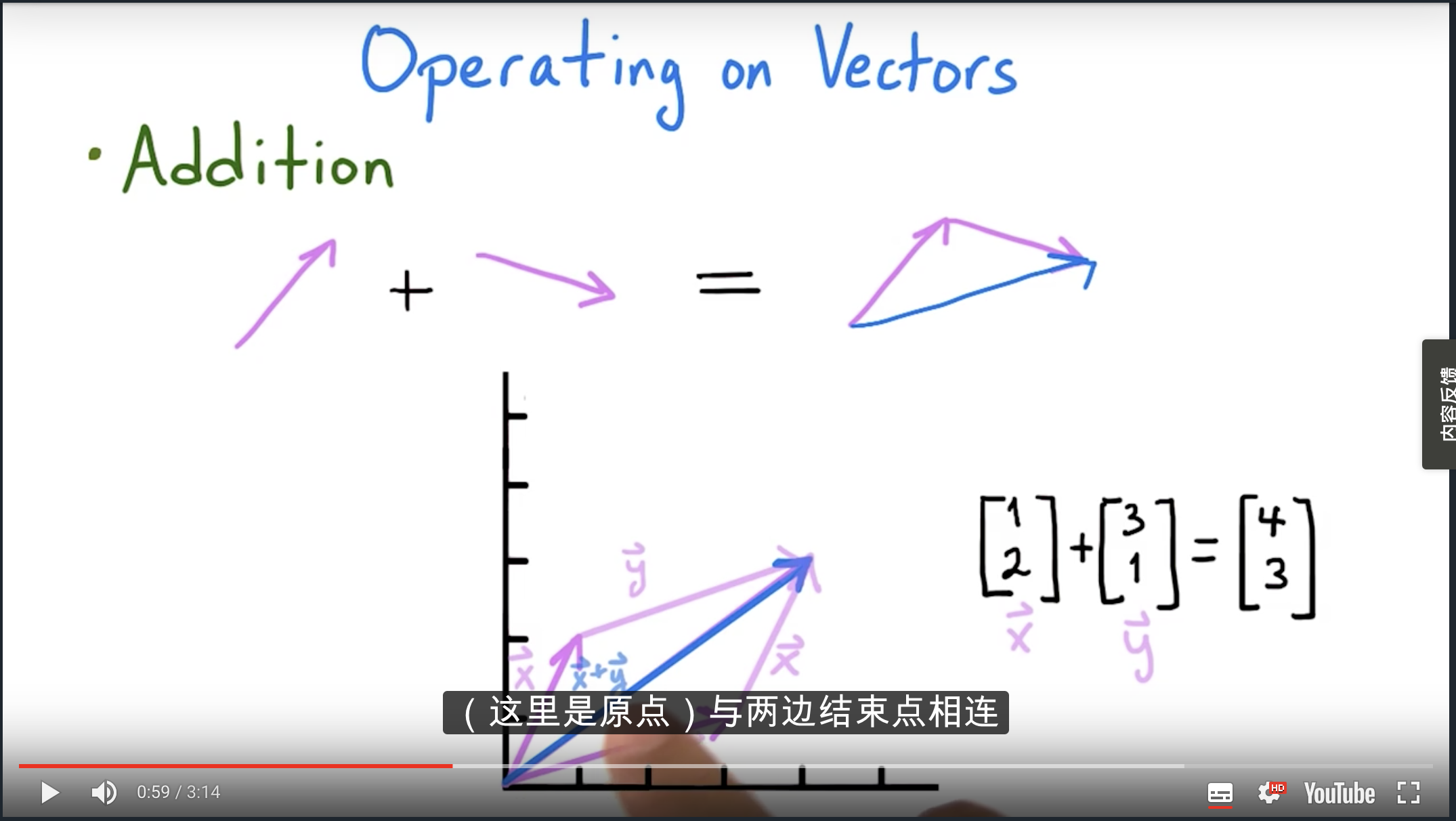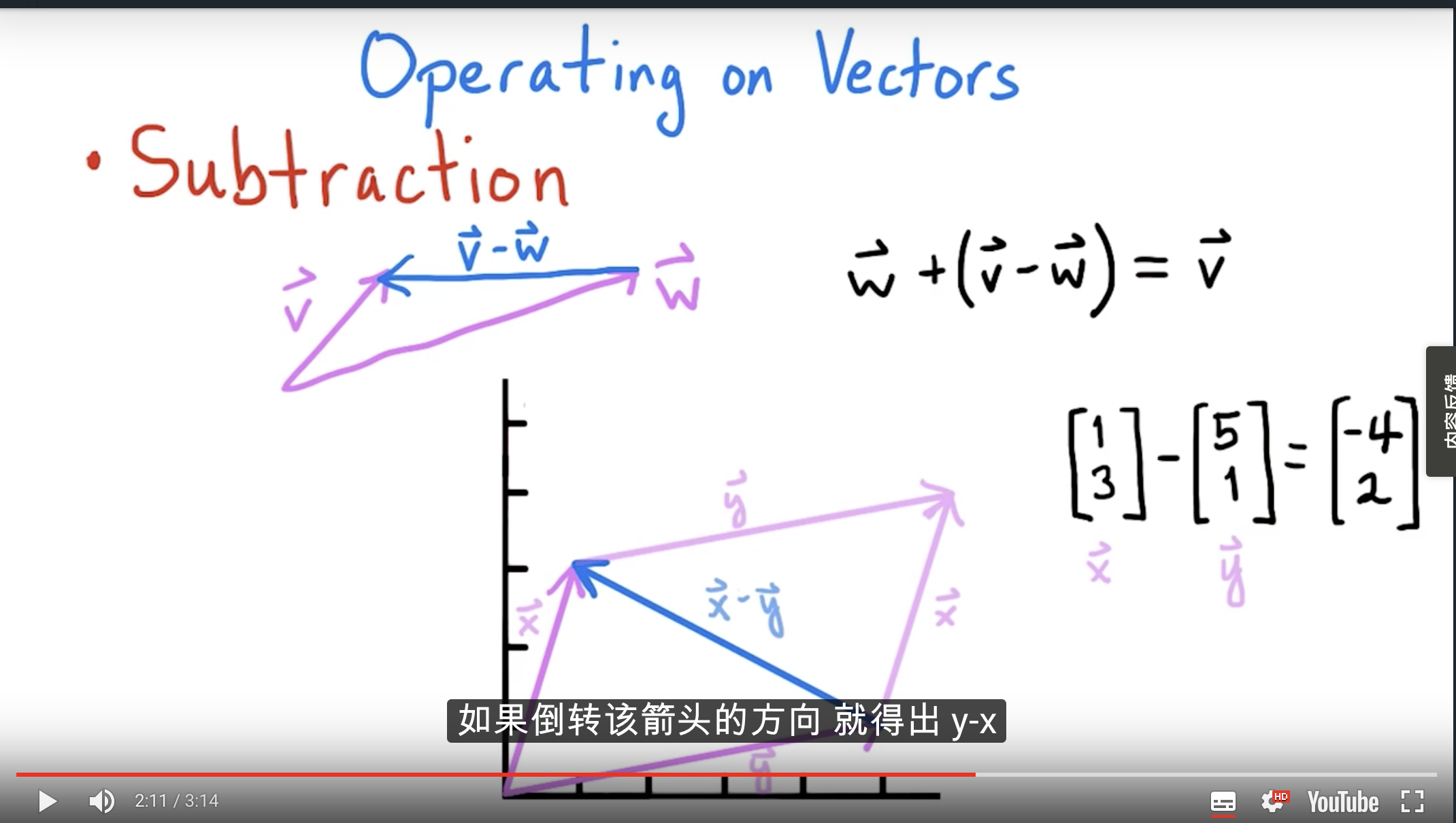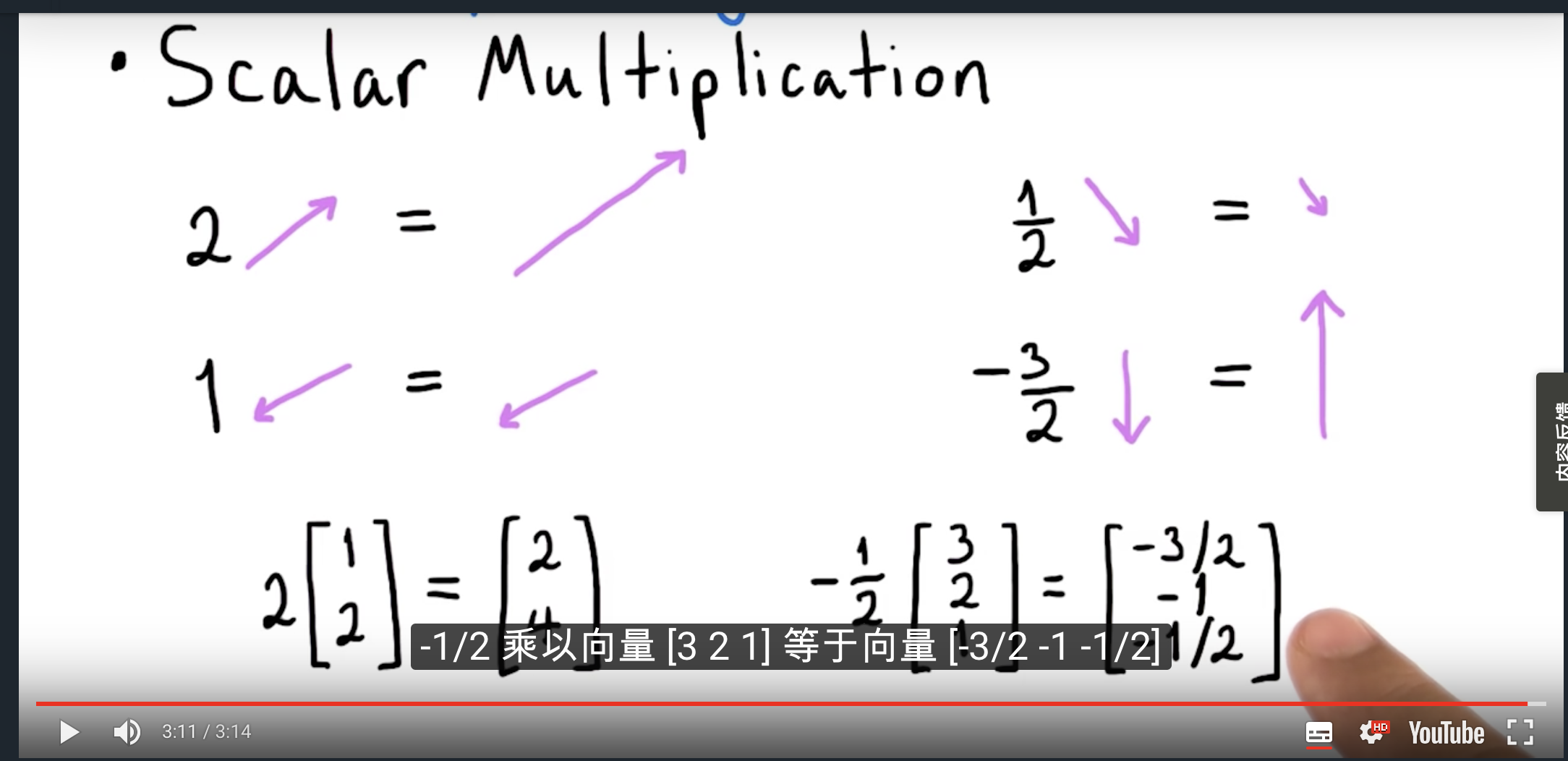展开全文•python
• 1、向量的相加\\乘\除 %矩阵的转置 a=[1,5,6]; b=[5,3,1]; %相加 c=a+b %相减 e=a-b %相乘 f=a'*b %与矩阵相乘 g=a*3 1、矩阵相加 %矩阵的加法 a=[1,1,1;2,5,66;4,7,8] b=[2,2,2;3,0,0;7,0,1] c=a+b ...
• 物理中，力的合成其实就是向量的加法。比如，下面是两条小船在牵引一艘大船，合力可以通过平行四边形法则（右上）或者三角形法则（右下）计算得到： 三角形法则在计算多个向量相加的时候非常方便，所以下面着重...
• 1、矩阵的加减乘除求逆运算的概念： 　（1）矩阵概念  有m n个数排列成一个m行n 列，并括以方括弧（或圆括弧）的数表称为m行n 列矩阵。 　（2）矩阵加法：   （3）矩阵乘法： 　（4）矩阵的求逆运算 　...
• 熟悉复数的几种表达方式及其加减乘除运算规则；掌握正弦量的相量表示法、相量的性能及其运算方法；掌握复阻抗和复导纳的概念；学会用相量图进行正弦量的辅助分析；正确理解正弦交流电路中几种功率的分析
• ## Tensorflow-加减乘除

万次阅读 2017-10-31 08:58:50
学习了一下TensorFlow的几个简单运算，虽说简单但对于初学者来说还是有几个值得注意的问题； 1.声明矩阵的时候，维度是完全需要[]控制的，用我的理解就是每增加一个维度就要用一个[]包括起其中内容；可通过下面代码...TensorFlow
• 实现的简单的Verilog进行十进制加减乘除，适合初学者。Verilog
• 如果要实现链式+法,必须运算完后,把对象返回继续+法操作(同链式调用) return self class UserScope(Scope): scope_api = ['blueprint_v1_users.api+get_user'] def __init__(self): self + Scope() class ...
• 先看一个程序：def main(args: Array[String]): Unit = { val str = "spark,hadoop,scala,java" val st = str.split(",") val map = new HashMap[String,Int]() var ps = "%" var sum = 17spark
• 重载运算符的方法（实现对应的方法） ... # 只要实现此方法即可重载+法操作(对象对象) def __add__(self, other): return self.a + other.b class Test001(Test): a = 3 b = 5 def __...
• 矩阵也存在加减乘除 矩阵就是填满数字的表格，一般用大写字母表示，关于矩阵很重要的一点是，它不是一个自然的概念，它是数值的一种表示方法，矩阵的运算也是人为约定的(人造的规则，完全可以采用不同的方法) 假设...
• 向量运算向量向量 向量大小、长度、模 标量与向量的乘法 标准化向量 向量的加法和减法 距离公式 向量点乘 向量投影 向量叉乘 C++语言标量与向量的乘法除法 放大一个向量的长度（乘以一个标量）缩放一个向量...
• 文章目录 创建tensor 直接创建 从numpy中获得数据 创建特定的tensor 根据数值要求： 根据矩阵要求: 随机采用生成: 基本运算加减乘除 乘法 对数运算： 幂函数： 指数运算 截断函数 对比操作： python number的获取 ...
• 纪念五个小时的Debug 第一次写博客，没啥话好说的，放代码得 #include<iostream> using namespace std; template<typename T> class vector { private: T* v; int len; ... v = n...c++
• 回顾流运算符和加减运算符的重载。 重点掌握赋值运算符的重载。 void operator=（const Vector&v）； #include <iostream> using namespace std; /* *作者:youshen_wwd *时间：2020/5/14 *题目：设向量X=...c++
• 例：let “I = I + 1” 或 let i=i+1 算术运算符指的是可以在程序中实现、乘、除等数学运算的运算符。Shell中常用的数学运算符如下所示。 +：对两个变量做加法。 -：对两个变量做减法。 *：对两个变量做乘法...linux
• # 两个数据的操作 lst1=np.array([10,20,30,40]) lst2=np.array([4,3,2,1]) print(lst1+lst2) # 和 print(lst1-lst2) # 差 print(lst1*lst2) # 乘 print(lst1/lst2) # 除 print(lst1**2) # 平方 ...print...
• 数组运算指的是数组对应元素之间的运算，也称作点运算，而等下讲到的矩阵的乘法、除法以及乘方那些都是有特殊的数学含义，和数组相对应元素的运算不一样，所以会在数组乘法、除法和乘方的运算符前个点表示点运算。...matlab 强化学习
• int型的加减乘除运算，四个函数指针放在一个vector向量中，通过vector向量调用函数。 输入字符串，将字符串拆解为两个整数和运算符，通过运算符种类调用不同的vector元素。 #include "mylib.h" #include "fact.h...
• 除了正常的加减乘除以外，向量的最常见的三个运算是点积、叉积、正交基。 对于向量的乘法和除法要做一下说明，因为除发的效率要远低于乘法，因此会将除法尽可能的化为乘法来实现。比如我们要对向量缩放一半，则可以...正交基
• 本文介绍了向量的定义、向量的模、负向量、单位向量、零向量以及向量加减法的三种实现方法。人工智能 数学 线性代数
• 《统计建模与R软件》薛毅 第2章 R软件的使用 2.5 多维数组和矩阵 总结： 2.5.3 数组的四则运算 ...可以对数组之间进行四则...数组的加、减法运算和数乘运算满足原矩阵运算的性质，数组即数乘数加减，都是数组及数...
• 高精度计算的方法： 1：先用字符串进行输入，并用向量对其逆序转化位数字 2：模拟四则运算法则对其进行计算； 3：倒序输出 以下是高精度计算的代码，希望对小伙伴有所帮助！！！ 函数1：计算高精度加法；( a + b ) ...算法...